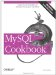# Categorizing Non-Categorical Data

7.13.1 Problem

You need to perform a summary on a set of values that are mostly unique and do not categorize well.

7.13.2 Solution

Use an expression to group the values into categories.

7.13.3 Discussion

One important application for grouping by expression results is to provide categories for values that are not particularly categorical. This is useful because GROUP BY works best for columns with repetitive values. For example, you might attempt to perform a population analysis by grouping records in the states table using values in the pop column. As it happens, that would not work very well, due to the high number of distinct values in the column. In fact, they're all distinct, as the following query shows:

```mysql> SELECT COUNT(pop), COUNT(DISTINCT pop) FROM states;
+------------+---------------------+
| COUNT(pop) | COUNT(DISTINCT pop) |
+------------+---------------------+
| 50 | 50 |
+------------+---------------------+```

In situations like this, where values do not group nicely into a small number of sets, you can use a transformation that forces them into categories. First, determine the population range:

```mysql> SELECT MIN(pop), MAX(pop) FROM states;
+----------+----------+
| MIN(pop) | MAX(pop) |
+----------+----------+
| 453588 | 29760021 |
+----------+----------+```

We can see from that result that if we divide the pop values by five million, they'll group into six categoriesa reasonable number. (The category ranges will be 1 to 5,000,000; 5,000,001 to 10,000,000; and so forth.) To put each population value in the proper category, divide by five million and use the integer result:

```mysql> SELECT FLOOR(pop/5000000) AS 'population (millions)',
-> COUNT(*) AS 'number of states'
-> FROM states GROUP BY 1;
+-----------------------+------------------+
| population (millions) | number of states |
+-----------------------+------------------+
| 0 | 35 |
| 1 | 8 |
| 2 | 4 |
| 3 | 2 |
| 5 | 1 |
+-----------------------+------------------+```

Hm. That's not quite right. The expression groups the population values into a small number of categories, all right, but doesn't report the category values properly. Let's try multiplying the FLOOR( ) results by five:

```mysql> SELECT FLOOR(pop/5000000)*5 AS 'population (millions)',
-> COUNT(*) AS 'number of states'
-> FROM states GROUP BY 1;
+-----------------------+------------------+
| population (millions) | number of states |
+-----------------------+------------------+
| 0 | 35 |
| 5 | 8 |
| 10 | 4 |
| 15 | 2 |
| 25 | 1 |
+-----------------------+------------------+```

Hey, that still isn't correct! The maximum state population was 29,760,021, which should go into a category for 30 million, not one for 25 million. The problem is that the category-producing expression groups values toward the lower bound of each category. To group values toward the upper bound instead, use the following little trick. For categories of size n, you can place a value x into the proper category using the following expression:

`FLOOR((x+(n-1))/n)`

So the final form of our query looks like this:

```mysql> SELECT FLOOR((pop+4999999)/5000000)*5 AS 'population (millions)',
-> COUNT(*) AS 'number of states'
-> FROM states GROUP BY 1;
+-----------------------+------------------+
| population (millions) | number of states |
+-----------------------+------------------+
| 5 | 35 |
| 10 | 8 |
| 15 | 4 |
| 20 | 2 |
| 30 | 1 |
+-----------------------+------------------+```

The result shows clearly that the majority of U.S. states have a population of five million or less.

This technique works for all kinds of numeric values. For example, you can group mail table records into categories of 100,000 bytes as follows:

```mysql> SELECT FLOOR((size+99999)/100000) AS 'size (100KB)',
-> COUNT(*) AS 'number of messages'
-> FROM mail GROUP BY 1;
+--------------+--------------------+
| size (100KB) | number of messages |
+--------------+--------------------+
| 1 | 13 |
| 2 | 1 |
| 10 | 1 |
| 24 | 1 |
+--------------+--------------------+```

In some instances, it may be more appropriate to categorize groups on a logarithmic scale. For example, the state population values can be treated that way as follows:

```mysql> SELECT FLOOR(LOG10(pop)) AS 'log10(population)',
-> COUNT(*) AS 'number of states'
-> FROM states GROUP BY 1;
+-------------------+------------------+
| log10(population) | number of states |
+-------------------+------------------+
| 5 | 7 |
| 6 | 36 |
| 7 | 7 |
+-------------------+------------------+```

## How Repetitive Is a Set of Values?

To assess how much repetition is present in a set of values, use the ratio of COUNT(DISTINCT) and COUNT( ). If all values are unique, both counts will be the same and the ratio will be 1. This is the case for the t values in the mail table and the pop values in the states table:

```mysql> SELECT COUNT(DISTINCT t) / COUNT(t) FROM mail;
+------------------------------+
| COUNT(DISTINCT t) / COUNT(t) |
+------------------------------+
| 1.00 |
+------------------------------+
mysql> SELECT COUNT(DISTINCT pop) / COUNT(pop) FROM states;
+----------------------------------+
| COUNT(DISTINCT pop) / COUNT(pop) |
+----------------------------------+
| 1.00 |
+----------------------------------+```

For a more repetitive set of values, COUNT(DISTINCT) will be less than COUNT( ) and the ratio will be smaller:

```mysql> SELECT COUNT(DISTINCT name) / COUNT(name) FROM driver_log;
+------------------------------------+
| COUNT(DISTINCT name) / COUNT(name) |
+------------------------------------+
| 0.30 |
+------------------------------------+```

What's the practical use for this ratio? A result close to zero indicates a high degree of repetition, which means the values will group into a small number of categories naturally. A result of 1 or close to it indicates many unique values, with the consequence that GROUP BY won't be very efficient for grouping the values into categories. (That is, there will be a lot of categories, relative to the number of values.) This tells you that to generate a summary, you'll probably find it necessary to impose an artificial categorization on the values, using the techniques described in this section.MySQL Cookbook
ISBN: 059652708X
EAN: 2147483647
Year: 2005
Pages: 412
Authors: Paul DuBois